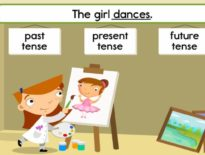# 6th-8th Grade Math Learning Activity: The Area of a Circle

We all know the area of a circle is πr2, but what is the math behind that equation? This interactive video will explain the logic behind the formula.

This interactive activity is embedded in our Math courses.

COURSE OVERVIEW

Students take a broader look at computational and problem-solving skills while learning the language of algebra. Students translate word phrases and sentences into mathematical expressions; analyze geometric figures; solve problems involving percentages, ratios, and proportions; graph different kinds of equations and inequalities; calculate statistical measures and probabilities; apply the Pythagorean theorem; and explain strategies for solving real-world problems. Online lessons provide demonstrations of key concepts, as well as interactive problems with contextual feedback. A textbook supplements the online material. Students who take Pre-Algebra are expected to have mastered the skills and concepts presented in the K12 Fundamentals of Geometry and Algebra course (or equivalent).

If you like this activity you can find an online school in your state.

Request a free catalog of K-12 Courses

Request the Scope & Sequence for a course

## 6th-8th Grade Math Learning Activity: The Area of …